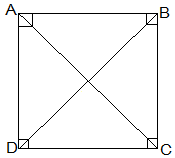Guru

# ABCD is a rectangle in which diagonal AC bisects ∠A as well as ∠C. Show that: (ii) Diagonal BD bisects ∠B as well as ∠D. Q.8 (2)

• 0

Yesterday i was doing the question from class 9th ncert book of math of Quadrilaterals chapter of exercise 8.1  What is the easiest way for solving it because i was not able to do this question please help me for solving this question of 8.  ABCD is a rectangle in which diagonal AC bisects ∠A as well as ∠C. Show that:  (ii) Diagonal BD bisects ∠B as well as ∠D.

Share

1.(ii) In ΔBCD,

BC = CD

⇒ ∠CDB = ∠CBD (Angles opposite to equal sides are equal)

also, ∠CDB = ∠ABD (Alternate interior angles)

⇒ ∠CBD = ∠ABD

Thus, BD bisects ∠B

Now,